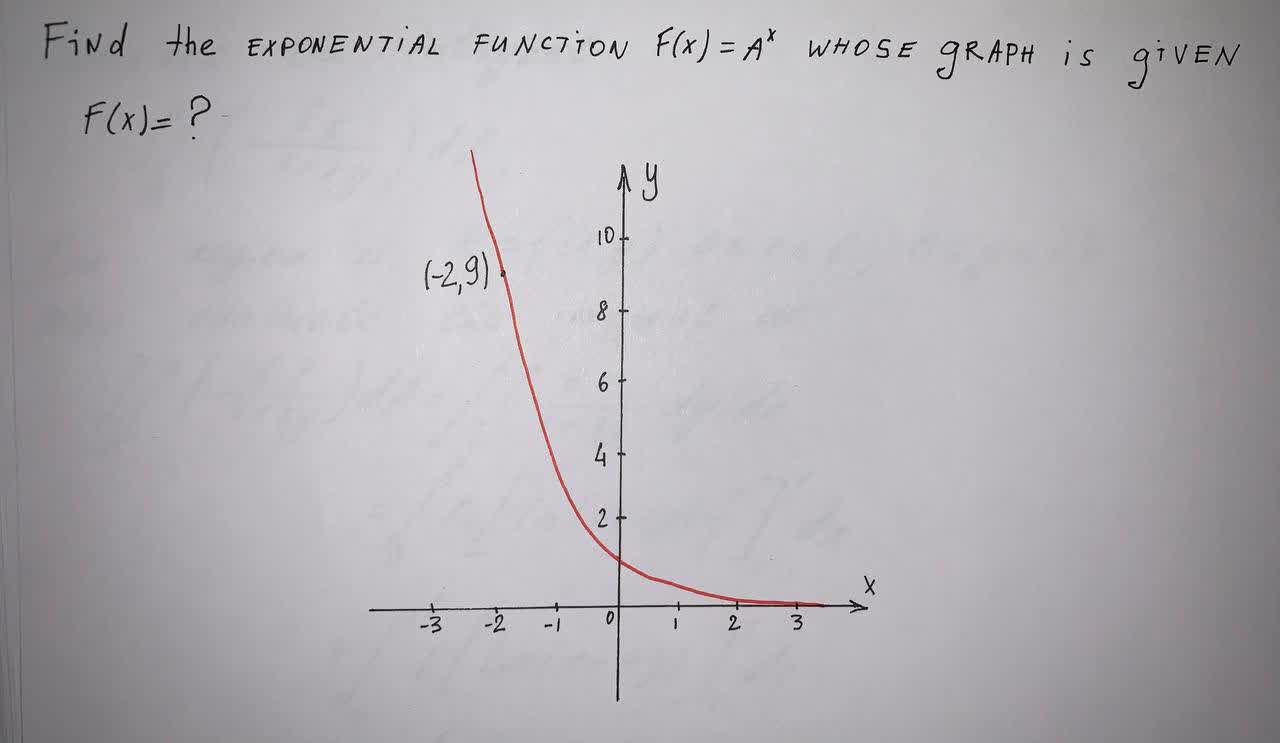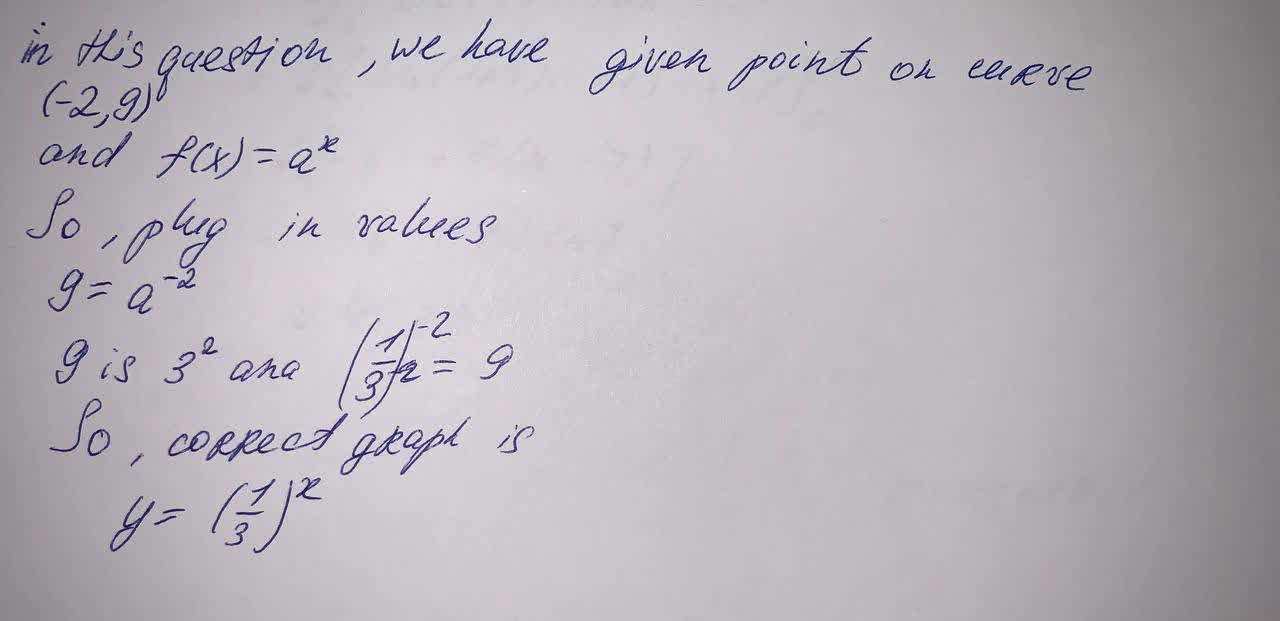Question# Find The Exponential Function f(x) = a^x Whose Graph Is Given.

Exponential models
ANSWEREDFind The Exponential Function $$\displaystyle{f{{\left({x}\right)}}}={a}^{{x}}$$ Whose Graph Is Given.